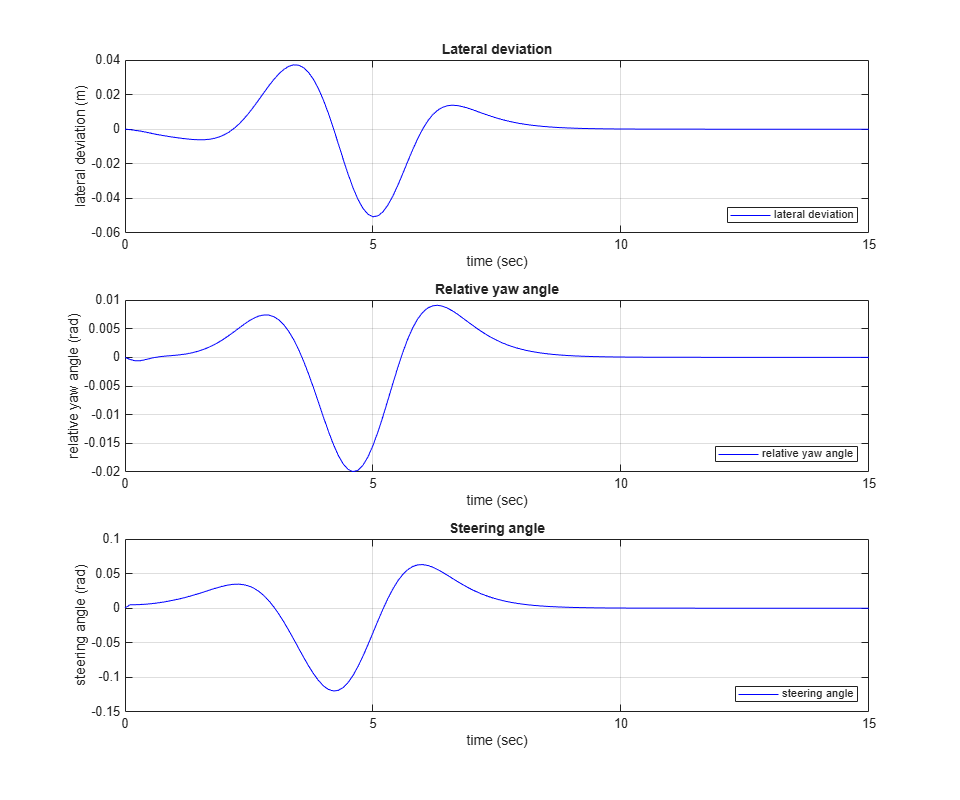# Lane Keeping Assist System Using Model Predictive Control

This example shows how to use the Lane Keeping Assist System block in Simulink® and demonstrates the control objectives and constraints of this block.

### Lane Keeping Assist System

A vehicle (ego car) equipped with a lane-keeping assist (LKA) system has a sensor, such as camera, that measures the lateral deviation and relative yaw angle between the centerline of a lane and the ego car. The sensor also measures the current lane curvature and curvature derivative. Depending on the curve length that the sensor can view, the curvature in front of the ego car can be calculated from the current curvature and curvature derivative.

The LKA system keeps the ego car travelling along the centerline of the lanes on the road by adjusting the front steering angle of the ego car. The goal for lane keeping control is to drive both lateral deviation and relative yaw angle close to zero.### Simulink Model for Ego Car

The dynamics for ego car are modeled in Simulink. Open the Simulink model.

mdl = 'mpcLKAsystem'; open_system(mdl)Define the sample time, Ts, and simulation duration, T, in seconds.

Ts = 0.1; T = 15; 

To describe the lateral vehicle dynamics, this example uses a bicycle model with the following parameters:

• m is the total vehicle mass (kg).

• Iz is the yaw moment of inertia of the vehicle (mNs^2).

• lf is the longitudinal distance from the center of gravity to the front tires (m).

• lr is the longitudinal distance from center of gravity to the rear tires (m).

• Cf is the cornering stiffness of the front tires (N/rad).

• Cr is the cornering stiffness of the rear tires (N/rad).

m = 1575; Iz = 2875; lf = 1.2; lr = 1.6; Cf = 19000; Cr = 33000; 

You can represent the lateral vehicle dynamics using a linear time-invariant (LTI) system with the following state, input, and output variables. The initial conditions for the state variables are assumed to be zero.

• State variables: Lateral velocityand yaw angle rate• Input variable: Front steering angle• Output variables: Same as state variables

In this example, the longitudinal vehicle dynamics are separated from the lateral vehicle dynamics. Therefore, the longitudinal velocity is assumed to be constant. In practice, the longitudinal velocity can vary. The Lane Keeping Assist System block uses adaptive MPC to adjust the model of the lateral dynamics accordingly.

% Specify the longitudinal velocity in m/s. Vx = 15; 

Specify a state-space model, G(s), of the lateral vehicle dynamics.

A = [-(2*Cf+2*Cr)/m/Vx, -Vx-(2*Cf*lf-2*Cr*lr)/m/Vx;... -(2*Cf*lf-2*Cr*lr)/Iz/Vx, -(2*Cf*lf^2+2*Cr*lr^2)/Iz/Vx]; B = [2*Cf/m, 2*Cf*lf/Iz]'; C = eye(2); G = ss(A,B,C,0); 

### Sensor Dynamics and Curvature Previewer

In this example, the Sensor Dynamics block outputs the lateral deviation and relative yaw angle. The dynamics for relative yaw angle are, wheredenotes the curvature. The dynamics for lateral deviation are.

The Curvature Previewer block outputs the previewed curvature with a look-ahead time of one second. Therefore, given a sample time, the prediction horizon 10 steps. The curvature used in this example is calculated based on trajectories for a double lane change maneuver.

Specify the prediction horizon and obtain the previewed curvature.

PredictionHorizon = 10; time = 0:0.1:15; md = getCurvature(Vx,time); 

### Configuration of the Lane Keeping Assist System Block

The LKA system is modeled in Simulink using the Lane Keeping Assist System block. The inputs to the LKA system block are:

• Previewed curvature (from lane detections)

• Ego longitudinal velocity

• Lateral deviation (from lane detections)

• Relative yaw angle (from lane detections)

The output of the LKA system is the front steering angle of the ego car. Considering the physical limitations of the ego car, the steering angle is constrained to the range [-0.5,0.5] rad/s.

u_min = -0.5; u_max = 0.5; 

For this example, the default parameters of the Lane Keeping Assist System block match the simulation parameters. If your simulation parameters differ from the default values, update the block parameters accordingly.

### Simulation Analysis

Run the model.

sim(mdl) 
 Assuming no disturbance added to measured output channel #1. -->Assuming output disturbance added to measured output channel #2 is integrated white noise. -->The "Model.Noise" property of the "mpc" object is empty. Assuming white noise on each measured output channel. 

Plot the simulation results.

mpcLKAplot(logsout)The lateral deviation and the relative yaw angle both converge to zero. That is, the ego car follows the road closely based on the previewed curvature.

bdclose(mdl)